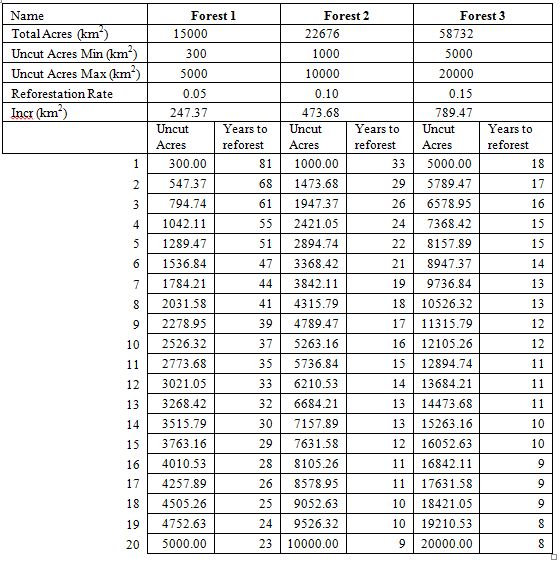GNG1106 C Programming Editing Services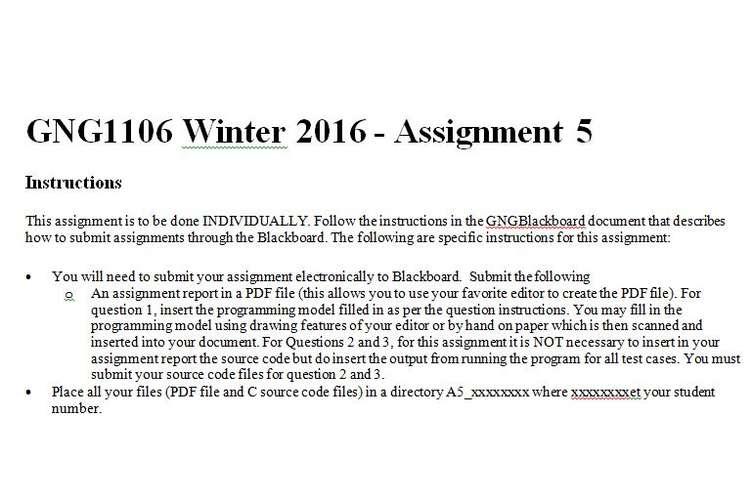Instructions

This assignment is to be done INDIVIDUALLY. Follow the instructions in the GNGBlackboard document that describes how to submit assignments through the Blackboard. The following are specific instructions for this assignment:
You will need to submit your assignment electronically to Blackboard.  Submit the following

• An assignment report in a PDF file (this allows you to use your favorite editor to create the PDF file). For question 1, insert the programming model filled in as per the question instructions. You may fill in the programming model using drawing features of your editor or by hand on paper which is then scanned and inserted into your document. For Questions 2 and 3, for this assignment it is NOT necessary to insert in your assignment report the source code but do insert the output from running the program for all test cases. You must submit your source code files for question 2 and 3.
• Place all your files (PDF file and C source code files) in a directory A5_xxxxxxxx where xxxxxxxx et your student number.
• Zip your PDF document and the C source files in a zip file with the name A5_xxxxxx.zip where xxxxxx is your student number and submit the zip file before the assignment deadline.
• The questions are provided in both PDF and Word files. You may use the Word file (please remove the questions) to enter your answers in the document. An rtf file is also provided so that you may edit the file with a word processor other than Word.  Do save your file as a PDF file for submission.
• Do start the assignment soon and do not wait until the last minute. You will be more efficient with a number of smaller efforts over a few weeks before the deadline than one large effort just before the deadline.

Marking Scheme

• Question 1: 5 marks
• Question 2: 10 marks
• Question 3: 15 marks

Question 1

The following programming model contains in its program memory the indicated C program composed of a main function. You will be showing how the working memory is used during the execution of this function. Show how the given C program affects the contents of the working memory:

• Show how the array and variables occupy space in the working memory.
• Show how the variables and arrays are initialized and updated by the loop.
• Show the values are assigned the variable and elements in the array by the assignment instructions. Be sure to show all values that are assigned and replaced. Record successive assignments to variables/parameters as follows (the ? shows an unknown value):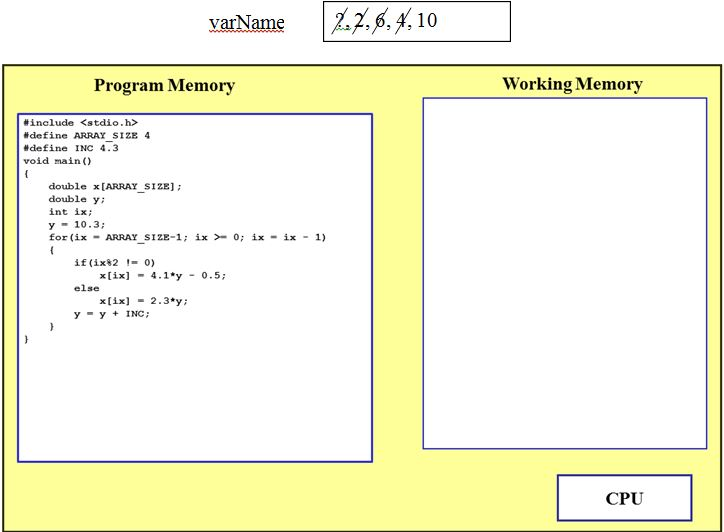Question 2

Ever wonder how the standard C math functions make their calculations. This question lets you explore how to compute ex for any value of x. The following infinite series gives the value of ex (where x is positive, i.e. greater or equal to 0):

Such series serves as a basis for calculating ex in a program (i.e. in a function). Of course a program has to limit the number of terms for computing the value (it is impossible to compute an infinite number of terms). Thus the above equation becomes: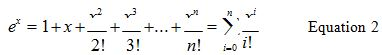Develop software that meets the following requirements:

• Prompts the user for the value of x and the number of terms in the series used to compute ex.

o  Check that the number of terms given by the user is positive.

• Computes the value of ex  using the above series and displays the results to the user.
• Prompts the user to quit the program with the message “Do you wish to quit (y/n)? “, and read in a character. Repeat the above steps if the user enters ‘y’ and stop the program if the user answers ‘n’. Keep prompting the user if an invalid answer is entered.
• Print the message “Program terminated” when the program terminates. Respect the following guidelines:
• Have the main function call a separate function, getInput, to get input values from the user. Recall that you have two options to have a called value return values to the calling function: the function can return a single value and the calling function can pass an address to the called function.
• Have the main function call a separate function, exponent, to compute the value of ex.
• Given that the powers of x in the numerator and the factorial in the denominator will become very large in a very few terms, calculating the values of each term separately will quickly reach the limit of the computer.
• Use a strategy where the value of a term is calculated from the previous term. If we number the terms using i starting at 0 (and the term t0 = 1), then the term ti can be computed from the previous term ti-1  as follows: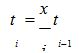• The above computation does not involve the same large numbers as the numerator/denominator of the terms in Equation 1.
• When x is negative, the result is calculated as 1/e-x where the series can be used to compute the denominator.

The main function uses the following steps:

• A loop to control termination of the program is part of the main function.
• In the loop the functions getInput and exponent are called and results displayed to the user before prompting to terminate the program.
• Recall that fflush function should be called before reading in a character input from the user.
• Use %g to display the value returned by exponent.

1. The source code to your program in a C file (DO NOT insert the source code into your assignment document, PDF file).
2. Insert the output for the following test cases into your assignment document. Include testing of bad values for N and the answer when prompting to quit.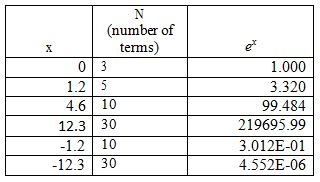Note that the values in the above table are calculated using Excel; the software may not give identical results, particularly for low value of N. The following shows a sample output that tests the above values as well as improper input.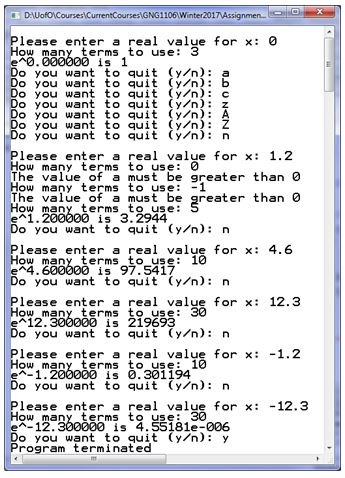Question 3

Timber Regrowth
On problem in timber management is to determine how much of an area to leave uncut so that the harvested area is reforested in a certain period of time.  It is assumed that reforestation take place at a known constant rate, r¸ per year, depending on climate and soil conditions. A reforestation equation expresses this growth as a function of the amount of timber standing and the reforestation rate.

Ai+1  = Ai   + rAi

where     i represents the year, 0 after harvesting is completed, and 1, 2, 3 represents the number of years after harvesting (thus A0 represents the area uncut after harvesting is completed, and A1, A2, A3, … represents the forest area after years 1, 2, 3, …).

r is the reforestation rate expressed as a fraction (for example 0.05 means that the forested area is increases by 5% at the end of a year).

You are responsible for developing a software tool that supports analysis of reforestation.  Given (from the user)

• the number of total acres,
• a minimum value for acres uncut,
• a maximum value for acres uncut,
• and reforestation rate,

show a table that increments the values of forest area by 20 (from the minimum to the maximum) and for each of the values the total number of years it takes to totally reforest the area.  The following shows a sample interaction with the user.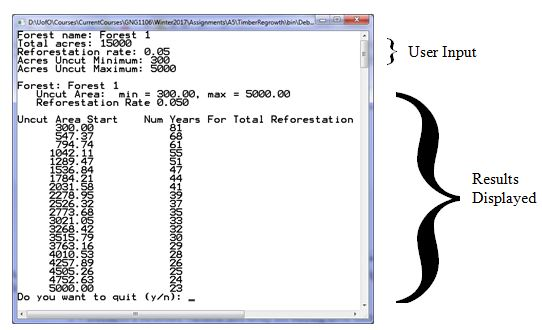A template software project has been provided (see file TimberRegrowth.zip). Complete the project using the following guidelines:

• Function: main
• Declares a structure variable and array for storing table data (use symbolic constants where possible).
• Use a loop to repeat the process (get user input, fill in the table data and print a table) until the user wishes to quit.
• Call the other function to get user input, fill in the table data, and display the table data.
• Function getForestInput

• This function uses a pointer to fill in the structure variable declared in main (see the function parameter).
• Data input by the user must be checked so that
• Total Acres, Acres uncut minimum and maximum must be greater than zero.
• The reforestation rate must be between 0 and 1 (exclusive, i.e. cannot be 0 or 1).

• Function calculateTableData
• Note that although the symbolic constants NUM_ROWS and NUM_COLS are available, parameters are used to define the dimension of the 2D array. This makes the function general and more easily ported to other software projects.
• Nested loops are required to complete this function.
• The first loop (outer loop) is used fill in the table and repeated by varying a row index variable from 0 to nRows-1 (i.e the number of rows).
• The value of uncut acres is also varied at each iteration of the loop from the minimum to maximum value given by the user. That is, the value of uncut acres start at the minimum value (given in the FOREST structure) and incremented up to the maximum value. The increment value is calculated by taking the difference of the minimum and maximum value and dividing it by nRows-1 (take the time to think of why nRows-1 and not nRows).
• This inner loop is used to compute the total years for reforestation for each value of uncut acres. The forested area is initialized to the uncut area and increased according to the reforestation rate as long as the reforested area is less than the total area.  The number of years is incremented each time the forested area is increased.
• After the number of years for reforestation is determined using the inner loop. A row in the array is updated with the values of the uncut acres and the number of years it takes to reforest the total area.
• Function displayTable
• This function first displays the forest information system (see the sample output).
• It then displays the contents of the array in table form (see the sample output).
• Please respect the output format such as the number of digits in the fraction part of the real numbers. Note that the years are displayed as integers although they are stored in the array as real values.